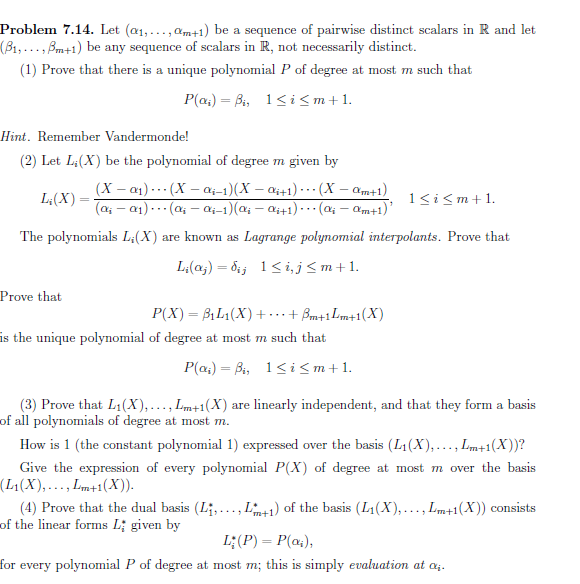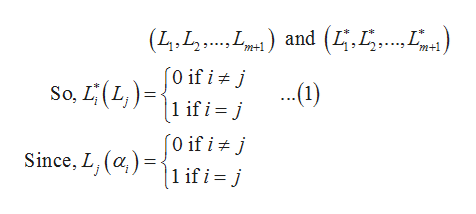# Problem 7.14. Let (æ1,...,am+1) be a sequence of pairwise distinct scalars in R and let(B1,...,Bm+1) be any sequence of scalars in R, not necessarily distinct.(1) Prove that there is a unique polynomial P of degree at most m such thatP(a4) Bi, 1i

Question
3 views

Hello, kindly assist me with the solution to Q4. I will appreciate it if you provide a very detailed solution, thankshelp_outlineImage TranscriptioncloseProblem 7.14. Let (æ1,...,am+1) be a sequence of pairwise distinct scalars in R and let (B1,...,Bm+1) be any sequence of scalars in R, not necessarily distinct. (1) Prove that there is a unique polynomial P of degree at most m such that P(a4) Bi, 1i
check_circle

Step 1

Basis and Dual basis are given as,help_outlineImage Transcriptioncloseand (4.5. (4.L. 0 if i j .1) if i= j m+1 So, LL 1 if i = j [0 if i Since, L, ()1 if i = j fullscreen
Step 2

Hence, equation (1) can also be written as

Step 3

Above equation can also be writt...

### Want to see the full answer?

See Solution

#### Want to see this answer and more?

Solutions are written by subject experts who are available 24/7. Questions are typically answered within 1 hour.*

See Solution
*Response times may vary by subject and question.
Tagged in

### Math